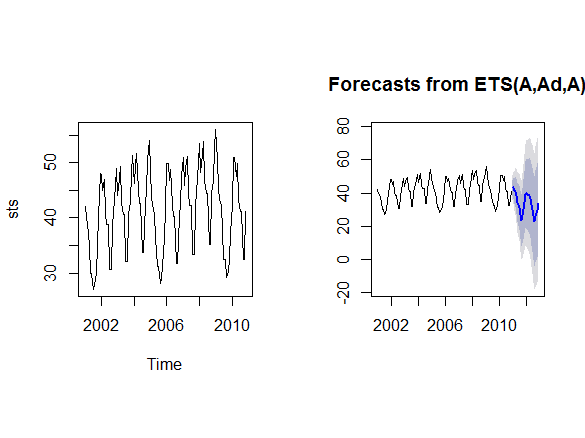# R - Forecasting

## Approaches to Forecasting

• ARIMA (AutoRegresive Integrated Moving Average)
• ETS (Exponential smoothing state space model)

We will discuss how those methods work and how to use them.

## Forecast package overview

 # Install Libraries
install.packages("forecast")
library("forecast")

rawdata$Date <- as.Date(rawdata$Date)
plot(rawdata)

# Using build in ts object
sts <- ts(rawdata$Sales,start=2001,frequency=12) plot(sts) #par(mfrow = c(2, 2)) # Forecast using ETS method fc.ets = forecast(sts) plot(fc.ets) plot(fc.ets$residuals)
plot(fc.etsfitted) # Forecast using ARIMA method ar = auto.arima(sts) ar fc.arima = forecast(ar) fc.arima plot(fc.arima) accuracy(fc.ets) accuracy(fc.arima)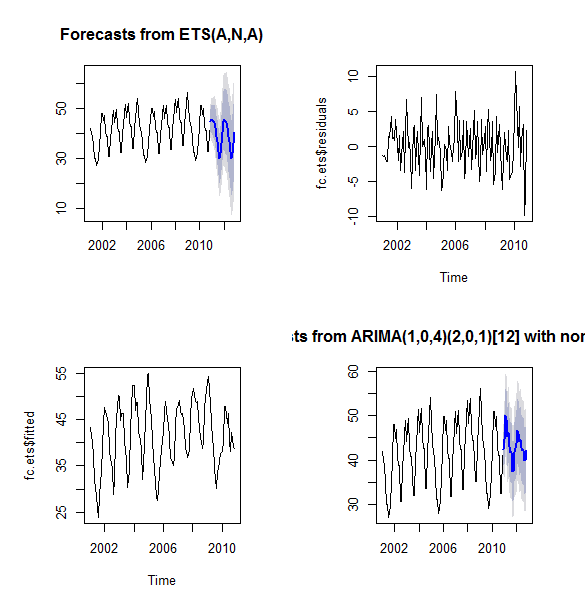## Exponential Smoothing Names • AKA: exponentially weighted moving average (EWMA) • Equivalent to ARIMA (0,1,1) model with no constant term Used for • smoothed data for presentation • make forecasts • simple moving average: past observations are weighted equally • exponential smoothing: assigns exponentially decreasing weights over time Formula {xt} - raw data sequence {st} - output of the exponential smoothing algorithm (estimate of the next value of x) α - smoothing factor, 0 < α < 1.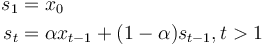\begin{align} s_1& = x_0\\ s_{t}& = \alpha x_{t-1} + (1-\alpha)s_{t-1}, t>1 \end{align} Choosing right α • no formal way of choosing α • statistical technique may be used to optimize the value of α (e.g. OLS) • the bigger the α the close it gets to naive forecasting (the same ports as original series with one period lag) ## Double Exponential Smoothing • Simple exponential smoothing does not do well when there is a trend (there will be always bias) • Double exponential smoothing is a group of methods dealing with the problem ### Holt-Winters double exponential smoothing Input • {xt} - raw data sequence of observations • t = 0 Model • {st} - smoothed value for time t • {bt} - best estimate of the trend at time t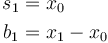\begin{align} s_1& = x_0\\ b_1& = x_1 - x_0\\ \end{align} And for t > 1 by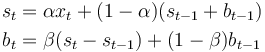\begin{align} s_{t}& = \alpha x_{t} + (1-\alpha)(s_{t-1} + b_{t-1})\\ b_{t}& = \beta (s_t - s_{t-1}) + (1-\beta)b_{t-1}\\ \end{align} where α is the data smoothing factor, 0 < α < 1, and β is the trend smoothing factor, 0 < β < 1. Output • Ft+m - an estimate of the value of x at time t+m, m>0 based on the raw data up to time t To forecast beyond xt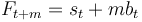\begin{align} F_{t+m}& = s_t + mb_t \end{align} ## Triple exponential smoothing • takes into account seasonal changes as well as trends • first suggested by Holt's student, Peter Winters, in 1960 Input • {xt} - raw data sequence of observations • t = 0 • L length a cycle of seasonal change The method calculates: • a trend line for the data • seasonal indices that weight the values in the trend line based on where that time point falls in the cycle of length L. • {st} represents the smoothed value of the constant part for time t. • {bt} represents the sequence of best estimates of the linear trend that are superimposed on the seasonal changes • {ct} is the sequence of seasonal correction factors • ct is the expected proportion of the predicted trend at any time t mod L in the cycle that the observations take on • To initialize the seasonal indices ct-L there must be at least one complete cycle in the data The output of the algorithm is again written as Ft+m, an estimate of the value of x at time t+m, m>0 based on the raw data up to time t. Triple exponential smoothing is given by the formulas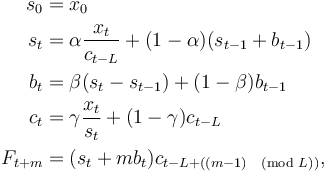\begin{align} s_0& = x_0\\ s_{t}& = \alpha \frac{x_{t}}{c_{t-L}} + (1-\alpha)(s_{t-1} + b_{t-1})\\ b_{t}& = \beta (s_t - s_{t-1}) + (1-\beta)b_{t-1}\\ c_{t}& = \gamma \frac{x_{t}}{s_{t}}+(1-\gamma)c_{t-L}\\ F_{t+m}& = (s_t + mb_t)c_{t-L+((m-1)\pmod L)}, \end{align} where α is the data smoothing factor, 0 < α < 1, β is the trend smoothing factor, 0 < β < 1, and γ is the seasonal change smoothing factor, 0 < γ < 1. The general formula for the initial trend estimate b0 is: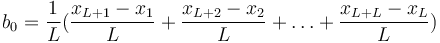\begin{align} b_0& = \frac{1}{L} (\frac{x_{L+1}-x_1}{L} + \frac{x_{L+2}-x_2}{L} + \ldots + \frac{x_{L+L}-x_L}{L}) \end{align} Setting the initial estimates for the seasonal indices ci for i = 1,2,...,L is a bit more involved. If N is the number of complete cycles present in your data, then: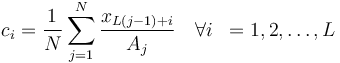\begin{align} \\ c_i& = \frac{1}{N} \sum_{j=1}^{N} \frac{x_{L(j-1)+i}}{A_j} \quad \forall i& = 1,2,\ldots,L \\ \end{align} where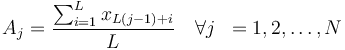\begin{align} A_j& = \frac{\sum_{i=1}^{L} x_{L(j-1)+i}}{L} \quad \forall j& = 1,2,\ldots,N \end{align} Note that Aj is the average value of x in the jth cycle of your data. ## ETS • Error, Trend, Seasonality ## Overriding parameters rawdata <- read.table("http://training-course-material.com/images/1/19/Sales-time-series.txt",h=T) rawdataDate <- as.Date(rawdata$Date) head(rawdata) plot(rawdata) # Using build in ts object sts <- ts(rawdata$Sales,start=2001,frequency=12)
plot(sts)

# Forecast using ETS method
model.ets = ets(sts,model="ANA")
fc.ets = forecast(model.ets)

model.ets1 = ets(sts,model="AAA",beta=0.2)
fc.ets1 = forecast(model.ets1)
plot(fc.ets1)

accuracy(fc.ets)
accuracy(fc.ets1)